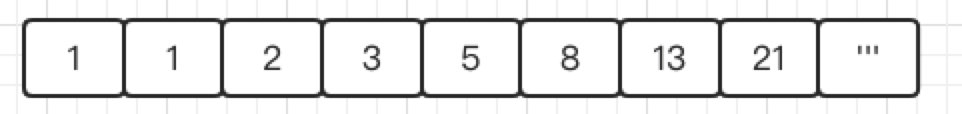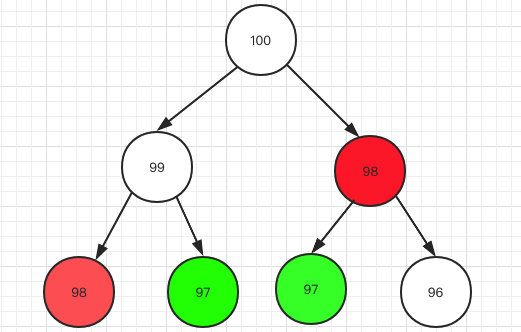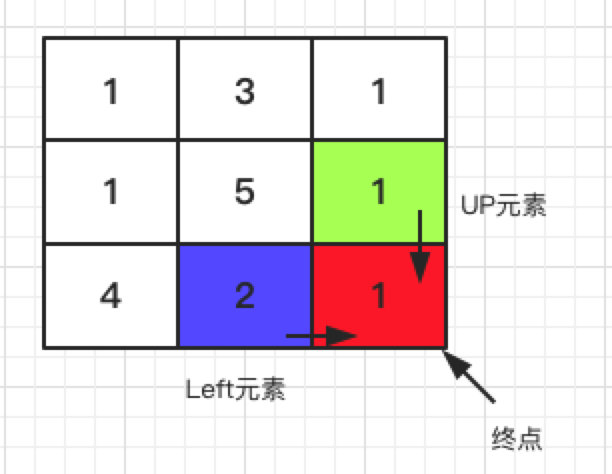# 安琪拉教鲁班学算法之动态规划

《安琪拉与面试官二三事》系列文章

《安琪拉教鲁班学算法》系列文章public class FibonacciSequence {
//求斐波拉契第n项
public int fibonacci(int n){
if(n == 1 || n == 2){
return 1;
}else{
return fibonacci(n-1) + fibonacci(n-2);
}
}
}public int fibonacciDP(int n){
if( n <= 2) {
return 1;
}
//保存前一项 以及 前前一项
int pre = 1, prepre = 1;
int index = 3;
int cur = pre + prepre;
while (index <= n){
//通过前一项和前前一项 得到当前值
cur = pre + prepre;
prepre = pre;
pre = cur;
index++;
}
return cur;
}


1. 需要求解的问题具有相似子问题，重点在相似二个字，例如上面的求第100个数需要第99 和 第 98 个数，求第 99 个数需要求第 98 和第 97个数，求解子问题具有相似性；
2. 需要求解的结果或最优结果由子问题的结果或最优结果推导而来，例如：F（n）= F（n-1）+ F（n-2）

1. 定义数组变量保存历史记录，避免重复计算，一般程序中都用dp[]定义，定义数组元素或变量的含义；
2. 找到数组元素之前的关系，这一步是最关键的，例如我们上面计算dp[n] 时，可通过dp[n-1] 和 dp[n-2] 获得；
3. 找到计算的起点以及值，例如上面斐波拉契数列的起点为 第一项 ：1，第二项：1，因为起点决定终点 😆。

1. 需要求解的问题具有相似子问题：爬100 级台阶的走法和爬99 级台阶走法都是跨1 、2 步求得，满足这个需求；
2. 需要求解的结果或最优结果由子问题的结果或最优结果推导而来，爬100 级台阶可以通过 爬完99 级台阶 + 跨1 步 这种走法 加 爬完98 级台阶 + 跨2步来完成，可以以此类推，满足特点；

• 第一步：定义数组或变量，我定义一个dp 分别保存 爬1级台阶走法，爬2级台阶走法 … 爬100级台阶的走法；

• 第二步：找元素的关系，爬到第n 级台阶的走法有二种：

1. 从第 n -1 级台阶 走 1 级上来
2. 从第 n -2 级台阶 走 2 级上来

因此总的走法为 dp[n] = dp[n-1] + dp[n-2]

• 第三步：找到计算的起点，

1. 当n = 1时，只有1 级台阶，只能1步跨上来，因此dp = 1；
2. 当n = 2时，有2级台阶，可以直接1步跨2级 或 一次跨1级走2步，以此dp = 2;

public void climbStairs() {
//1. 定义数组或变量
long[] dp = new long;
//3. 找到计算的起点
dp = 1;
dp = 2;

int index = 3;
while (index <= 100){
//2. 找元素的关系
dp[index] = dp[index-1] + dp[index-2];
index++;
}

System.out.println(dp);
}


/**
* 爬楼梯
* @param n 台阶数
* @return
*/
public long climbStairs(int n) {
if(n == 1 || n == 2){
return n;
}
//1. 定义数组或变量  3. 找到计算的起点
// dp_n_2 代表 dp[n-2]; dp_n_1代表 dp[n-1];
long dp_n_2 = 1;
long dp_n_1 = 2;

int index = 3;
long cur = dp_n_1 + dp_n_2;
while (index <= n){
//2. 找元素的关系
cur = dp_n_1 + dp_n_2;
dp_n_2 = dp_n_1;
dp_n_1 = cur;
index++;
}

return cur;
}


**说明：**每次只能向下或者向右移动一步。

输入:
[
[1,3,1],
[1,5,1],
[4,2,1]
]

**备注**：输入定义为 value[m][n] 二维数组 m 行 n 列，这里 m，n都为3


• 第一步：定义数组或变量，我定义一个dp[m][n] 的二维数组保存数组中任一位置到左上角起点的最小路径和；

• 第二步：找元素的关系，任意选取一个元素，看一下这个元素的值怎么求得，以选取终点为例，看下图：1. 因为条件限定了只能往右和往下走，如果要到终点，只能从UP元素或者Left元素过来，UP元素代表上方的元素，Left元素代表左侧元素，元素名是我自己给起的，为了方便说明；

2. 那终点元素的值 = Math.min( UP元素的最小路径和 , Left元素最小路径和 ) + 终点元素的值（这里是1）；

泛化成公式：dp[m] [n] = Math.min( dp[m-1] [n] ,dp[m] [n-1]) + value[m] [n];

所有动态规划的描述元素之间关系的公式都有个名字：状态转移方程

除终点元素，其他元素也符合这个规则，但是需要考虑边界问题，如果元素没有左元素或者上元素，只需要简单直接以左元素或者上元素中有的元素最小路径和 + 当前元素值。

• 第三步：找到计算的起点，

1. dp  是左上角第一个元素，作为起点，因为不能别的元素过来，因此 dp  = value ；

public int minPathSum(int[][] grid) {
//边界检查
if(grid == null || grid.length < 1 || grid.length < 1){
return 0;
}
//获取行数 和 列数
int m = grid.length;
int n = grid.length;
// 1. 定义二维数组 保存数组每个元素到起点的最小路径和
int[][] dp = new int[m][n];
for(int i = 0; i < m; i++){
for(int j = 0; j < n; j++){
//2. 找元素的关系 & 考虑边界条件
if(i == 0 && j == 0){
//第一个元素，没有上元素，也没有左元素
dp = grid;
}else if(i == 0){
//没有上元素，只能从左元素走过来
dp[i][j] = dp[i][j-1] + grid[i][j];
}else if(j == 0){
//没有左元素，只能从上元素走过来
dp[i][j] = dp[i-1][j] + grid[i][j];
}else{
//既有上元素，也有左元素
dp[i][j] = Math.min(dp[i][j-1], dp[i-1][j]) + grid[i][j];
}
}
}
//终点最小路径和
return dp[m-1][n-1];
}


1. 当前元素的dp 值只跟前二个元素dp 值有关，我只需要保存推导当前元素所需要的dp值就可以了，不需要申请完整的m * n 的额外空间；
2. 我还可以把边界检查的顺序优化一下，因为 i = 0 或 j = 0 属于特殊情况，检查的判断条件后置，从编译的角度优先对最有可能进入的条件分支进行判断。

public int minPathSum(int[][] grid) {
//省下 m * n 的额外空间，前提： grid 二维数组允许值被覆盖
for(int i = 0; i < grid.length; i++) {
for(int j = 0; j < grid.length; j++) {
//1. 最有可能的分支前置
if(i != 0 && j != 0){
grid[i][j] = Math.min(grid[i - 1][j], grid[i][j - 1]) + grid[i][j];
}
else if(i == 0)  grid[i][j] = grid[i][j - 1] + grid[i][j];
else if(j == 0)  grid[i][j] = grid[i - 1][j] + grid[i][j];
else continue;
}
}
return grid[grid.length - 1][grid.length - 1];
}


• 第一步：定义dp数组或变量，首先明确题目说每种硬币的数量是无限的，但是会给定一个固定的 amount 金额，我们需要用最少的硬币数凑出这个金额，因此可以定义dp[n] 为需要凑出金额n 所需要的最小硬币数；

• 第二步：找元素的关系，假设现在需要求dp（凑11块钱），现在手里有1，2，5块三种硬币，是不是10，9，6块钱都+ 1个硬币就可以凑齐11 块了（10块加1块，9块加2块，6块加5块）。那我们应该选10，9，6块中的哪一种呢？

就看凑齐10，9，6三种金额哪种方式用到的硬币数量最少，dp(11) = Math.min( dp(10), dp(9), dp(6) ) + 1;

我们试着开始推导一下状态转化方程：

1. dp[n] 为凑齐金额为n 所需要的最少硬币数量；
2. coins[] 为硬币种类数组，例如coins = [1, 2, 5] 代表硬币种类为 1块，2块，5块；
3. 假设现在dp ~ dp[n-1] 我们都求出来了，也就是凑齐0 ~ n-1元所需要的最少硬币数都知道了，现在dp[n] 怎么求？ 因为我们有1块，2块，5块钱，是不是很自然的想到凑齐n 元只需要从 dp[n-1] , dp[n-2], dp[n-5] 中选一个最小的 + 1。泛化状态转移方程：$dp[n] = \min_{j=0_\ldots n-1}dp(n-coin(j)) + 1$
• 第三步：找到计算的起点，dp = 0 (凑齐0元不需要硬币)

public int coinChange(int[] coins, int amount) {
//边界条件检查
if(amount < 0){
return -1;
}
if(amount == 0){
return 0;
}

//1. 定义dp数组，dp[n]代表凑齐n元所属最小硬币数
int[] dp = new int[amount+1];
Arrays.fill(dp, amount+1);
//3. 设置计算的起点
dp = 0;
for(int i = 0; i <= amount; i++){
//遍历所有硬币，试图找到最小硬币数的组合
for(int coin : coins){
if(i - coin >= 0){
dp[i] = Math.min(dp[i], dp[i-coin]+1);
}
}
}
return dp[amount] > amount ? -1 : dp[amount];
}


1. 需要求解的问题具有相似子问题，例如：按摩师前天约了，今天才能约，如果昨天约过了，今天不能约，因此第n天接受预约能达到的最长预约时长为 Math.max( 第n-2天最长预约时间 +今天的时间，第n-1天最长预约时间)，子问题同样依赖此公式;
2. 需要求解的结果或最优结果由子问题的结果或最优结果推导而来，这里是：dp（n）= Math.max(dp（n-1), dp（n-2）+value(今天))，预约不连续。

public int massage(int[] nums) {
if(nums == null || nums.length ==0){
return 0;
}
if(nums.length < 2){
return nums;
}
// 第一步：定义dp数组
int[] dp = new int[nums.length];
// 第三步：初始化起点
dp = nums;
dp = Math.max(dp, nums);
int i = 2;
while( i < nums.length){
// 第二步：状态转移方程
dp[i] = Math.max(dp[i-2]+nums[i], dp[i-1]);
i++;
}
return dp[nums.length-1];
}


public int rob(int[] nums) {
int len;
//边界检查
if(nums == null || (len = nums.length) == 0){
return 0;
}
if(len == 1){
return nums;
}
//第一步：定义dp变量
int dp_pre = nums;
int dp_cur = Math.max(dp_pre, nums);
int i = 2;
int tmp;
while(i < len){
tmp = dp_cur;
//状态转移方程
dp_cur = Math.max(dp_pre +nums[i] , dp_cur);
dp_pre = tmp;
i++;
}
return dp_cur;
}


class Solution {
public int rob(int[] nums) {
int len;
if(nums == null || (len=nums.length) == 0){
return 0;
}
if(len == 1){
return nums;
}
//不偷第一个 或  不偷最后一个
return Math.max(
myRob(Arrays.copyOfRange(nums, 0, nums.length-1)),
myRob(Arrays.copyOfRange(nums, 1, nums.length))
);
}

private int myRob(int[] nums){
int pre = 0;
int cur = 0;
int tmp;

for(int item : nums){
tmp = cur;
cur = Math.max(pre + item, cur);
pre = tmp;
}
return cur;
}
}


03-1610万+

#### 我说我不会算法，阿里把我挂了。02-111万+

#### 啥水平？谷歌程序员：我用东北方言编程

10-117万+

#### 经典中的经典算法:动态规划(详细解释,从入门到实践,逐步讲解)

09-29658

#### java实现寻找有向图的的闭环

03-199万+

#### 离职半年了，老东家又发 offer，回不回？

04-073万+

#### Synchronized关键字深析（小白慎入，深入jvm源码，两万字长文）

04-061927

#### 安琪拉教鲁班学算法之BFS和DFS

06-166万+

#### 六大算法之三：动态规划

04-061891

#### 安琪拉教鲁班学堆排序

04-303万+

#### 如何优雅的替换掉代码中的ifelse

03-252万+

#### 大学四年，我决定把Java学习过的书籍都分享一遍

05-1952

#### 300分钟搞定数据结构与算法课程学习5 ——动态规划

08-172355

#### 动态规划学习（一）：基本求解步骤

04-1763

#### 按摩师-动态规划

04-242万+

#### 从零开始学动态规划

05-03116

#### 算法设计与分析课程复习笔记7——动态规划

04-161332

#### 动态规划学习笔记

04-024173

#### 算法课笔记系列（四）—— 动态规划

03-0925

#### 数据结构与算法自学系列之动态规划（一）

03-264万+

#### “程序员数学不行，干啥都不行！”高级开发：90%都是瞎努力！

03-225万+

#### 男生更看重女生的身材脸蛋，还是思想？

09-111485

#### 【算法学习笔记】-动态规划

04-201968

#### 安琪拉教百里守约学并发编程之多线程基础

03-1269

#### 快速理解算法之动态规划

02-233万+

#### 程序员在家撸码的十大姿势

04-141409

#### 安琪拉教妲己分布式限流

05-294161

#### 王者荣耀-数模论文分享（虽然结果我自己都不信）

04-076万+

#### 超全Python图像处理讲解（多图预警）

03-101万+

#### 朋友被阿里面试官灵魂拷问，跑来求救。。。

02-0318万+

#### 为什么说程序员做外包没前途？©️2019 CSDN 皮肤主题: 技术黑板 设计师: CSDN官方博客点击重新获取扫码支付